# Silver Ratio Calculator

 Silver Ratio Calculator Enter Known Dimension: Width    A = Length    B =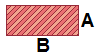Round to the nearest... whole number half      (0.5) quarter      (0.25) eighth      (0.125) tenth      (0.1) sixteenth      (0.0625) thirty-second      (0.03125) hundredth      (0.01) thousandth      (0.001) ten thousandth      (0.0001)

The silver ratio is an analog of the more well-known golden ratio. A silver rectangle has an aspect ratio of 1:1+√2, or approximately 1:2.41421356. The number 1 + √2 is not called the silver constant or silver number, as those terms are reserved for other numbers. The silver ratio is usually represented by the symbol δs.

The silver ratio arises in many mathematical and geometric expressions. For example, the continued fraction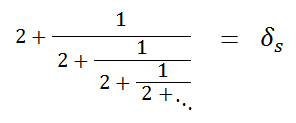converges to δs. The continued radicals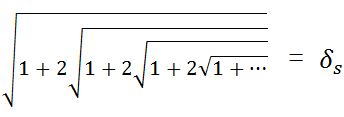and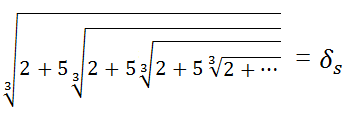also converge to δs. These arithmetic expressions can be resolved by solving the equations

x = 2 + 1/x,       x = √1+2x,      and   x = 2+5x.

The silver ratio also appears as the limiting ratio of solutions to the Pell equation 2x² ± 1 = y² For instance, the first several solution pairs are

(1, 1)     2x² - 1 = y²
(2, 3)     2x² + 1 = y²
(5, 7)     2x² - 1 = y²
(12, 17)     2x² + 1 = y²
(29, 41)     2x² - 1 = y²
(70, 99)     2x² + 1 = y²
(169, 239)     2x² - 1 = y²
(408, 577)     2x² + 1 = y²

If you take the ratio of consecutive y terms, you obtain

3/1 = 3
7/3 = 2.3
17/7 = 2.428571
41/17 = 2.411765
99/41 = 2.414634
239/99 = 2.41
577/239 = 2.414226

As you can see, the ratio converges to 1 + √2. You will also see the same pattern if you take the ratio of consecutive x terms.

To understand δs geometrically, suppose you have a rectangle whose sides are in a ratio of 1:δs. When you cut two perfect squares from the rectangle, the remaining rectangle is also a silver rectangle. If you repeat the process over and over, you obtain the pattern shown on the left: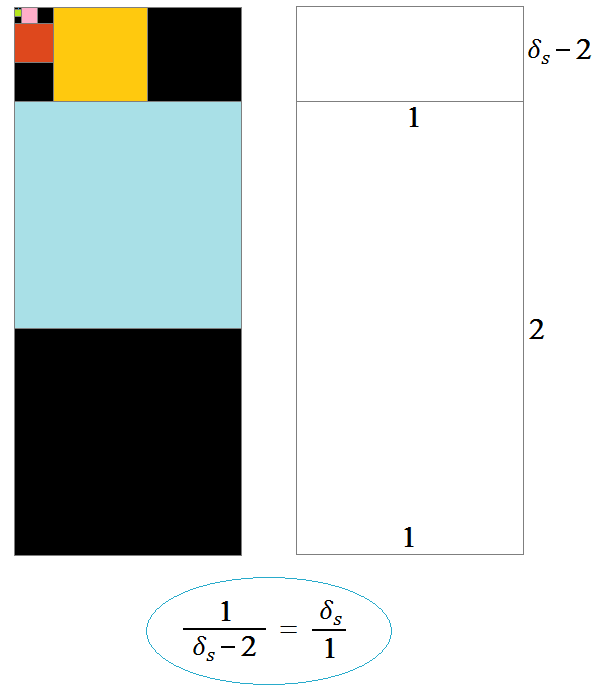The silver rectangle also appears within the octagon. If you form a rectangle from opposite sides of the octagon, it will have an aspect ratio of 1:δs.If you want to create a rectangle whose sides are in a ratio of 1:δs, and you know the length of one of the sides, you can quickly compute the length of the missing side with one of the following formulas. Here, "width" denotes the shorter side and "length" denotes the longer side.

width = length/δs
length = width × δs